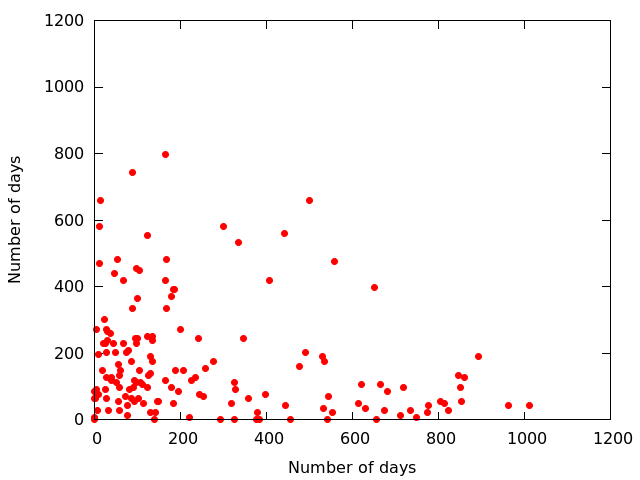> Marwan Ghanem, Clémence Magnien and Fabien TarissanOne of the main indicators of graph analyses is the centrality, which quantifies the importance of a node in the network. We are interested particularly in the closeness centrality, which relies on the shortest distance between the considered node and all other nodes in the network.

As the analyses of temporal graphs remain a challenge, one of the most common methods in temporal analyses is the aggregation of the dataset into a set of snapshots of equal duration. Analysing each snapshot separately as an aggregated graph and attributing a single value representing the whole duration of the snapshot, causes a loss of temporal information.

Therefore, we define an extension of the centrality closeness by defining a temporal distance from 2 nodes at a certain instance \$t\$ to be the duration of a shortest starting at t path connecting both nodes. Thus, we can evaluate a closeness centrality value that evolves over time.  The formal definition is given in the paper Time Evolution of the Importance of Nodes in dynamic Networks.

Here, we compare our method to the snapshot method using the Enron dataset : a company’s dataset that had 151 employees whom have exchanged approximately 250000 emails during a period of three years, each email representing a link in the network.

We calculate the closeness centrality using the two methods. We then use the scores of the ranking and compare the duration spent by each node in the 25 % highest ranks.

In the figure, each dot represents this duration calculated by our method on the x-axis and this duration calculated by the snapshot method on the y-axis. We can easily see how the nodes are scattered around the plot, rather than being close to the plot’s diagonal. In addition, we can see extreme cases where nodes are detected as highly important in one method and not in the other.

This comparison shows that while both methods are fit, each represents a different notion of importance

This entry was posted in Plots